SCERT AP 7th Class Maths Solutions Pdf Chapter 10 Construction of Triangles Ex 10.2 Textbook Exercise Questions and Answers.

## AP State Syllabus 7th Class Maths Solutions 10th Lesson Construction of Triangles Ex 10.2

Question 1.
Construct ∆ABC with measurements AB = 4.5 cm, BC = 6 cm and ∠B=75°
Given measurements of ∆ABC are AB = 4.5 cm, BC = 6 cm and ∠B = 75°.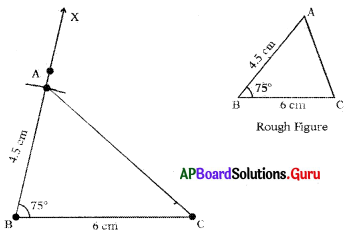Steps of Construction :

1. Draw a rough sketch of triangle and label it with given measurements.
2. Draw a line segment with BC = 6 cm.
3. Draw a ray $$\overrightarrow{\mathrm{BX}}$$ such that ∠CBX = 15°.
4. Draw an arc with centre B and radius 4.5 cm, to intersect $$\overrightarrow{\mathrm{BX}}$$ at point A.
Thus, required ∆ABC is constructed with the given measurements.Question 2.
Construct an isosceles triangle with measurements DE = 7 cm, EF = 7 cm and ∠E = 60°
Given measurements of ∆DEF are DE = 7 cm, EF = 7 cm and ∠E = 60°.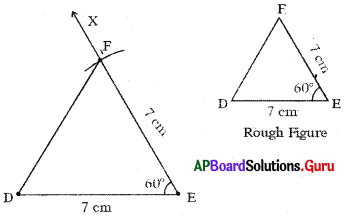Steps of Construction:

1. Draw a rough sketch of triangle and label it with given measurements.
2. Draw a line segment with DE = 7 cm.
3. Draw a ray $$\overrightarrow{\mathrm{EX}}$$ such that ∠DEX = 60°.
4. Draw an arc with centre E and radius 7 cm to intersect $$\overrightarrow{\mathrm{EX}}$$ at point F.
5. Join DF.
Hence, required ∆DEF is constructed with the given measurements.

Question 3.
Draw a triangle with,measurements ∠B = 50°, AB = 3 cm and AC = 4 cm.
Given measurements of a triangle are ∠B = 50°, AB = 3 cm and AC = 4cm..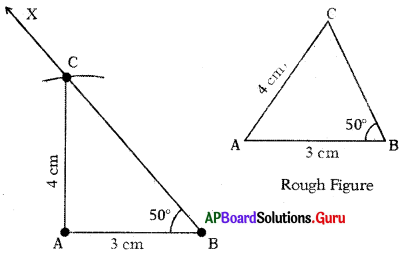Steps of Construction:

1. Draw a rough sketch of triangle and label it with given measurements.
2. Draw a line segment with AB = 3 cm.
3. Draw a ray $$\overrightarrow{\mathrm{BX}}$$ such that ∠ABX = 50°.
4. Draw an arc with centre A and radius 4 cm to intersect $$\overrightarrow{\mathrm{BX}}$$ at point C.
5. Join AC.
Hence, required ∆ABC is constructed with the given measurements.

Question 4.
Construct a right angled triangle in which, XY = 5 cm, XZ = 6 cm and right tingle at X.
Given measurements of ∆XYZ are XY = 5 cm, XZ = 6 cm and ZX = 90° (∠YXZ = 90°)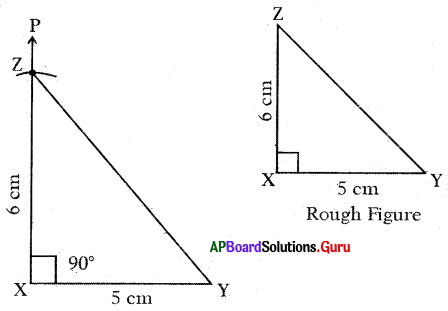Steps of Construction:

1. Draw a rough sketch of triangle arid label it with given measurements.
2. Draw a line segment with XY = 5 cm.
3. Draw a ray $$\overrightarrow{\mathrm{XP}}$$ such that ∠YXP = 90°.
4. Draw an arc with centre X and radius 6 cm to intersect XP at point Z.
5. Join YZ.
Hence, required triangle ∆XYZ is constructed with the given measurements.Question 5.
Construct a right angled with measurements ABC, ∠B = 90°, AB = 8 cm and AC = 10 cm.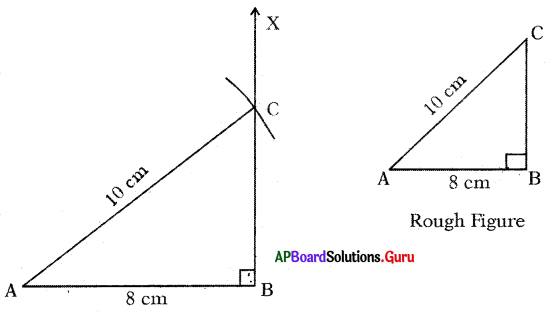3. Draw a ray $$\overrightarrow{\mathrm{BX}}$$ such that ∠ABX = 90°.
4. Draw an arc with centre A and radius 10 cm to intersect $$\overrightarrow{\mathrm{BX}}$$ at point C.